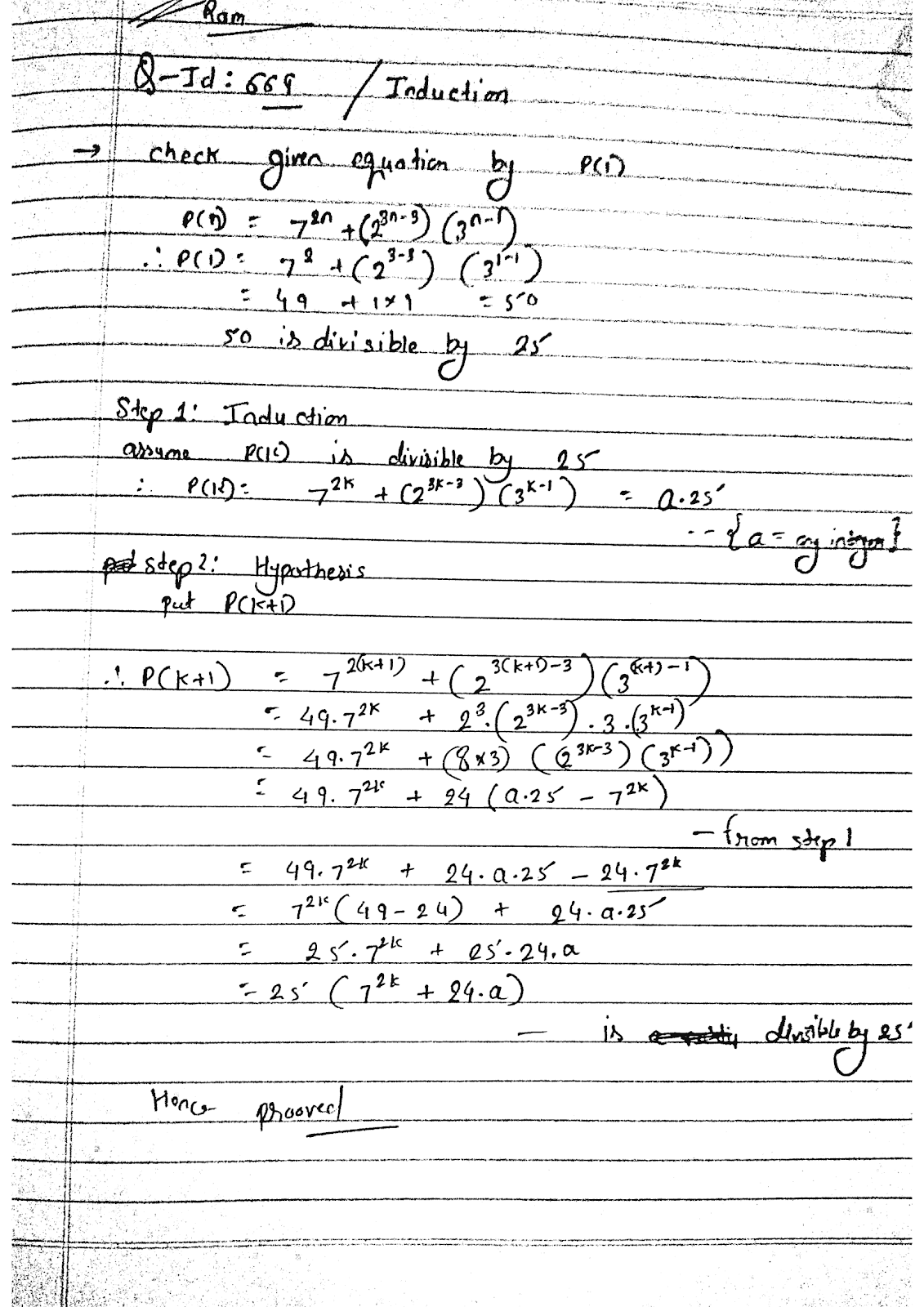# prove by using mathematical induction method that 7^2n+2^3n-3*3^n-1 is divisible by 25 for any natural number n

more_vert

prove by using mathematical induction method that 7^2n+2^3n-3*3^n-1 is divisible by 25 for any natural number n